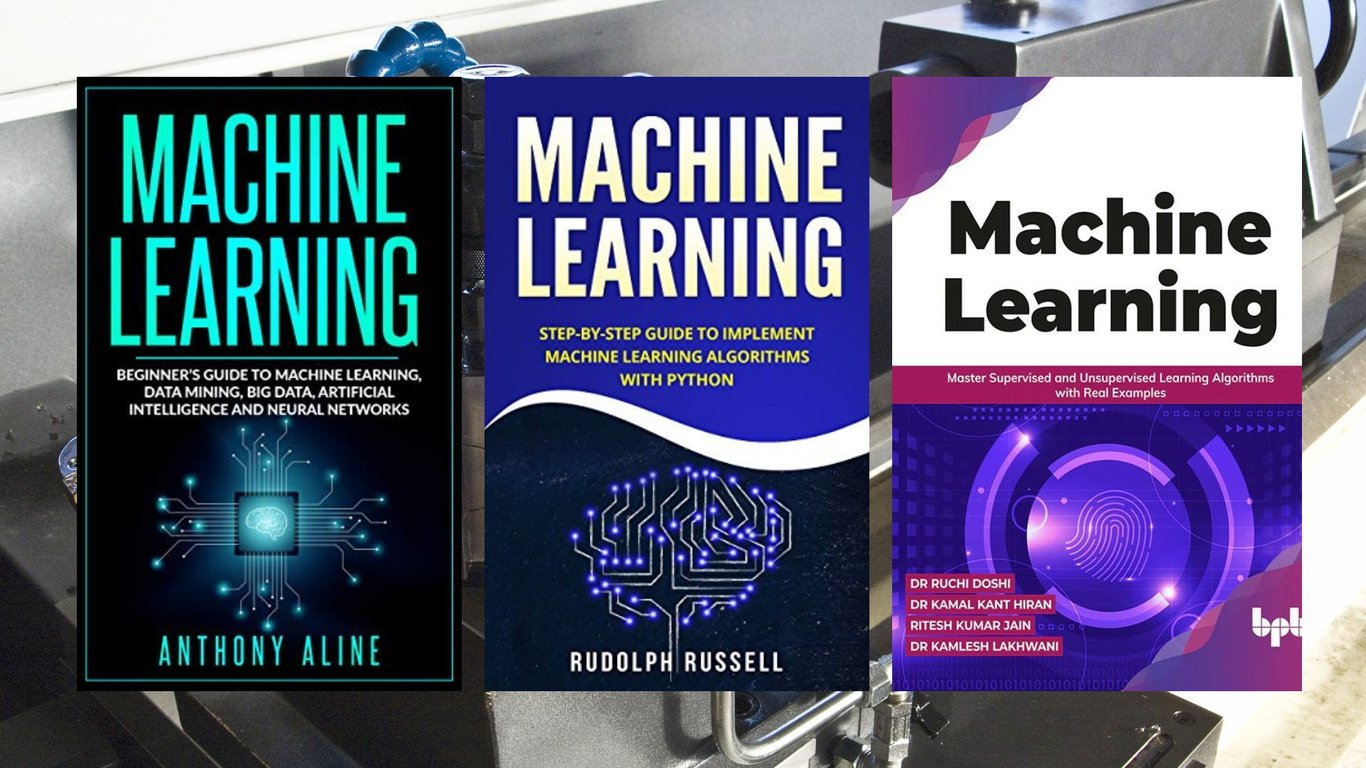# 6 Best Machine Learning Books For Beginners in 2023Are you intrigued by the world of machine learning but don't know where to start? Whether you're a complete beginner or have some basic knowledge, diving into the field of machine learning can be both exciting and overwhelming. That's why we've compiled a list of six highly recommended machine learning books for beginners in 2023. These books will provide you with a solid foundation, introducing you to the fundamental concepts, tools, and techniques in the world of machine learning.

## 1. Machine Learning for Absolute Beginners

If you're starting from scratch, "Machine Learning for Absolute Beginners" is the perfect starting point. This book provides a gentle introduction to the core concepts of machine learning without assuming any prior knowledge in the field. Through clear explanations and practical examples, you'll learn about supervised and unsupervised learning, feature engineering, model evaluation, and more. The book also includes hands-on exercises to help you grasp the concepts effectively.

## 2. The Hundred-Page Machine Learning Book

Short on time but eager to learn? "The Hundred-Page Machine Learning Book" is designed to give you a concise yet comprehensive overview of machine learning. Despite its brevity, this book covers a wide range of topics, from basic concepts to more advanced techniques. It's a great resource for those who want to quickly understand the key principles of machine learning without delving into excessive technical details.

## 3. Mathematics for Machine Learning

A strong foundation in mathematics is crucial for understanding the underlying principles of machine learning. "Mathematics for Machine Learning" focuses on the mathematical concepts that power various machine learning algorithms. This book introduces topics such as linear algebra, calculus, and probability theory in the context of machine learning applications. It's a valuable resource for beginners who want to deepen their understanding of the mathematical concepts behind the algorithms.

## 4. Hands-On Machine Learning with scikit-learn and TensorFlow

Looking to get your hands dirty with practical machine-learning projects? "Hands-On Machine Learning with scikit-learn and TensorFlow" is a hands-on guide that takes you through real-world examples using popular machine learning libraries. You'll learn to build and train machine learning models, evaluate their performance, and deploy them for various tasks. This book is perfect for beginners who want to learn by doing.

## 85. Linear Algebra and Optimization for Machine Learning

Linear algebra forms the backbone of many machine learning algorithms. "Linear Algebra and Optimization for Machine Learning" offers a beginner-friendly approach to understanding linear algebra and optimization techniques used in machine learning. The book explains concepts like vectors, matrices, eigenvalues, and gradient descent in a way that's accessible to those new to the field.

## 6. Deep Learning with PyTorch: A Practical Approach

Ready to dive into the exciting world of deep learning? "Deep Learning with PyTorch" provides a practical introduction to building and training neural networks using the PyTorch framework. This book covers the fundamentals of deep learning, including convolutional and recurrent networks, and guides you through hands-on projects to help solidify your understanding.

Whether you're intrigued by the theoretical aspects of machine learning or eager to jump into hands-on projects, these six books offer a well-rounded introduction to the field. Remember, the key to mastering machine learning is practice and persistence. Happy learning!

# Join AI & Bots mailing list

We won't spam. Pinky promise